Question

# Design a nondeterministic Turing Machine to accept the {ww | w ∈ {0,1}*} . Draw the...

Design a nondeterministic Turing Machine to accept the {ww | w ∈ {0,1}*} . Draw the transition diagram of the machine.

Non-deterministic turing machine

Consider the string "abaaba". this process have 2 stages . 1st stage is to find midpoint.2nd stage is to check whether the string is acceptable or not.

The below turing machine will accept a string in the form of "ww". for example consider the string "101101".

1st stage:

This TM will convert 0 into X and 1 into Y.

TM will scan the input string from left to right. when see the 1st '1' which will be converted into 'Y' and it will move to right upto the end of the string and the last '1' also be converted into 'Y'. now the string will look like Y0110Y. then it will move to Left most side and convert '0' into 'X' then move to right most side and convert last '0' into 'X'. now the string is YX11XY. it will do the similar action for the remaining string also. finally it will look like " YXYYXY ".

2nd stage :

After completing this conversion we have found the midpoint of the string. next step is to convert left part of the string into 0's and 1's. now the string in the form of 101YXY. after this again we need a conversion to check the string is acceptable or not. for that move to left and convert '1' into Y and move right convert Y into Blank (B). now the string is Y01BXY. then move to left and convert 0 into 'X' and move right convert X into B. do the same step for the entire string. now the string will be like YXYBBB. left part is in the form of X and Y and right part is in the form of B. here the string is accepted. in case any of the symbol is left unchanged in left or right part, the string will not be accepted by the turing machine. for example after the conversion if the string is like "YXY1BBB". here the string will be rejected.

Transition diagram: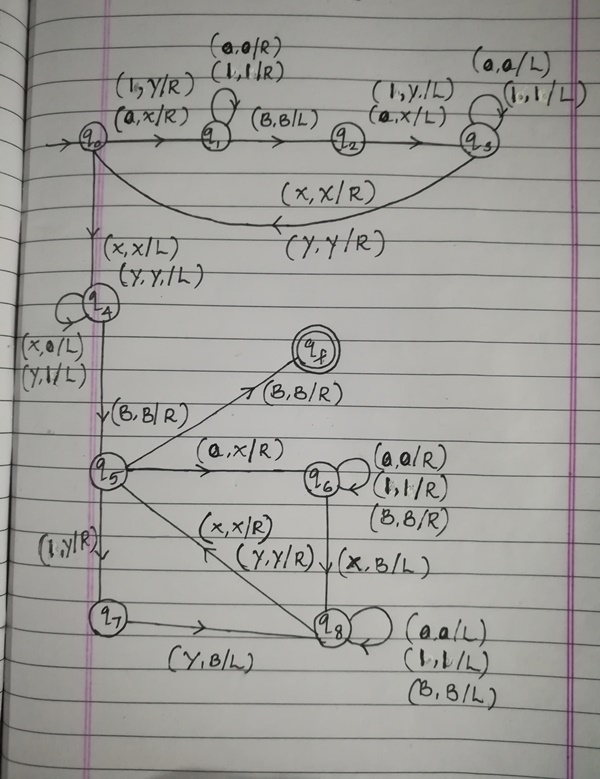**************END**************PLS GIVE ME UPVOTE*******************************

#### Earn Coins

Coins can be redeemed for fabulous gifts.

Similar Homework Help Questions
• ### 40 points) Please design a Turing machine T to recognize the union of the languages of two Turing...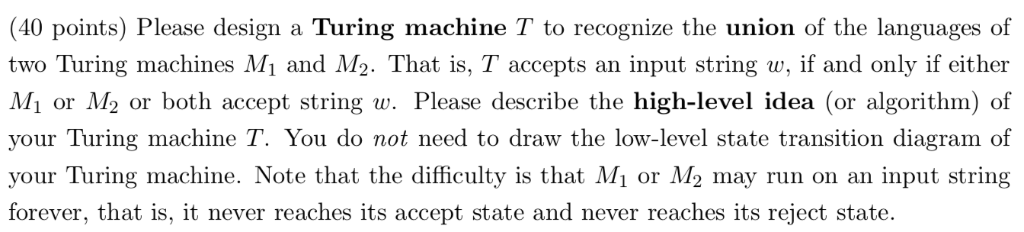40 points) Please design a Turing machine T to recognize the union of the languages of two Turing machines Mi and M2. That is, T accepts an input string w, if and only if either Mi or M2 or both accept string w. Please describe the high-level idea (or algorithm) of your Turing machine T. You do not need to draw the low-level state transition diagram of your Turing machine. Note that the difficulty is that Mi or M2 may...

• ### Design a Turing machine that recognizes the language L := {vSw : u, w E {0,1)" and u is a substri...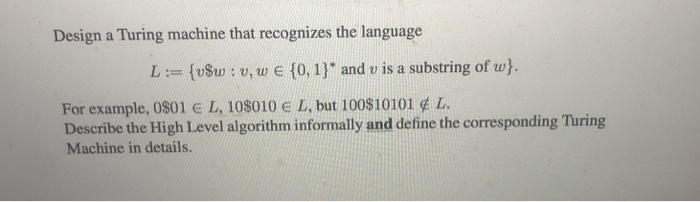Design a Turing machine that recognizes the language L := {vSw : u, w E {0,1)" and u is a substring of u For example, 0801 E L' 10\$010 E L, but i 00\$10101 ¢ L. Describe the High Level algorithm informally and define the corresponding Turing Machine in details. Design a Turing machine that recognizes the language L := {vSw : u, w E {0,1)" and u is a substring of u For example, 0801 E L' 10\$010 E...

• ### 1. (25 points) Turing Machine Design: Design a Turing machine Mi that operates on inputs that...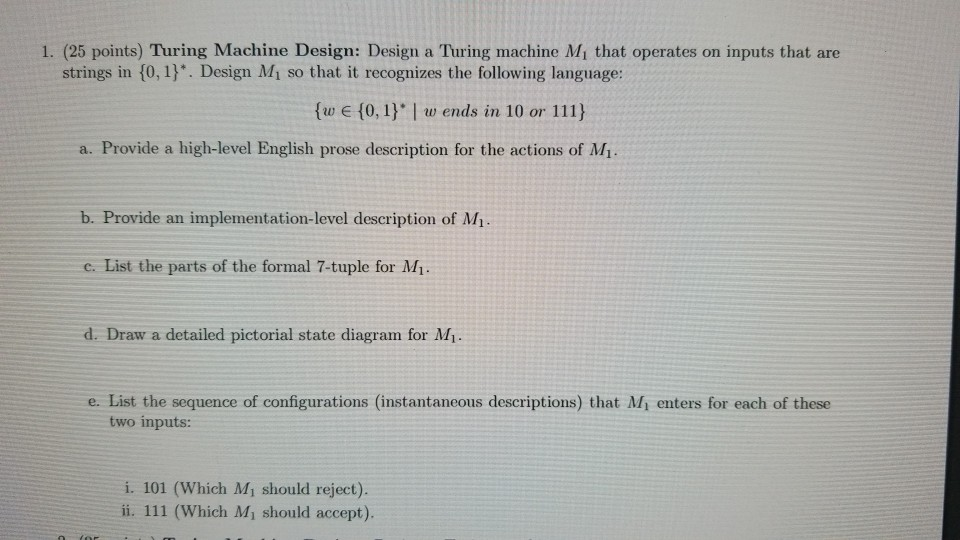1. (25 points) Turing Machine Design: Design a Turing machine Mi that operates on inputs that are strings in 10, 1). Design Mi so that it recognizes the following language: fw E (0.1)l w ends in 10 or 111) a. Provide a high-level English prose description for the actions of Mi b. Provide an implementation-level description of M. c. List the parts of the formal 7-tuple for M d. Draw a detailed pictorial state diagram for M1 e. List the...

• ### Describe the computational power of a single tape Turing machine compared to a nondeterministic single tape...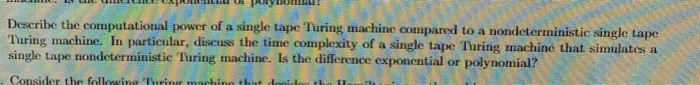Describe the computational power of a single tape Turing machine compared to a nondeterministic single tape Turing machine. In particular, discuss the time complexity of a single tape Turing machine that simulates a single tape nondeterministic Turing machine. Is the difference exponential or polynomial? . .

• ### 5. 20 Points Draw transition diagrams for standard Turing machines that accept the following languages. In...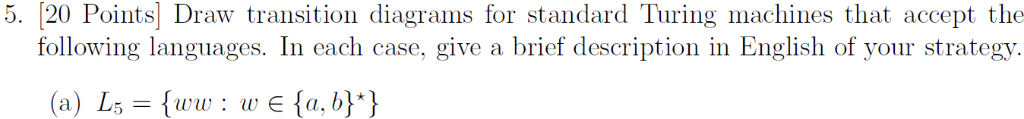5. 20 Points Draw transition diagrams for standard Turing machines that accept the following languages. In each case, give a brief description in English of your strategy. (a) Ls {ww w e {a, b}*} (b) Le wu w, и € fа, b}*, |u| 3D lul}

• ### Q6: Explain Nondeterministic Turing machines in detail. Also show how to convert non-deterministic Turing machine into...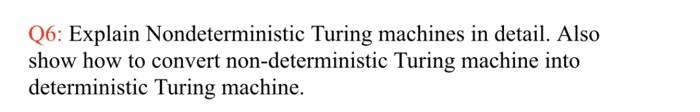Q6: Explain Nondeterministic Turing machines in detail. Also show how to convert non-deterministic Turing machine into deterministic Turing machine.

• ### State diagrams for Turing Machines. Suppose you are given a string w ∈ {0,1}* placed on a Turin...

State diagrams for Turing Machines. Suppose you are given a string w ∈ {0,1}* placed on a Turing Machine tape. Give the state diagram for the Turing Machine required to take the initial string, w, and replace it on the tape with a new string, w′. The new string, w′, is formed by shifting the entire input string one cell to the right. Suppose you are given a string w ∈ {0, 1}* placed on a Turing Machine tape. Give...

• ### Construct a Turing Machine (TM) that accepts the following language, defined over the alphabet Σ =...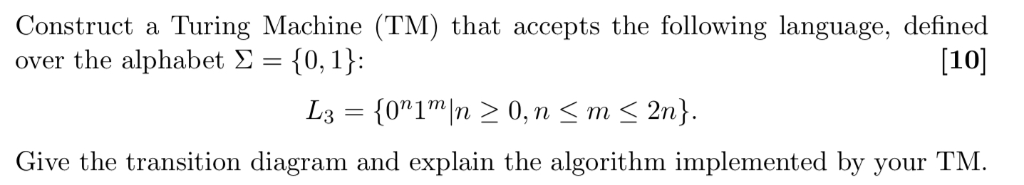Construct a Turing Machine (TM) that accepts the following language, defined over the alphabet Σ = {0,1): at accepts the tollowing language, define  Give the transition diagram and explain the algorithm implemented by your TM.

• ### Let L = {0^n 1^n | n ≥ 0}. Draw the state diagram of a Turing...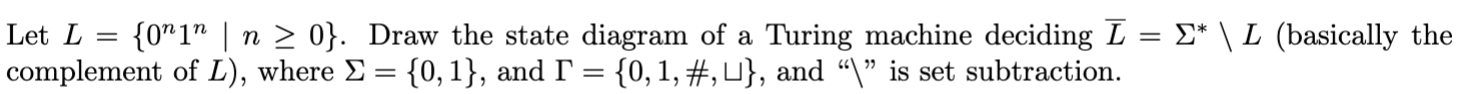Let L = {0^n 1^n | n ≥ 0}. Draw the state diagram of a Turing machine deciding L= Σ∗\L(basically the complement of L), where Σ = {0,1}, and Γ = {0,1,#,U}, and “\” is set subtraction. I understand that the complement of L will be {0^n 1^m | n=!m} U {(0 U 1)* 1 0 {0 U 1)*}. How should I draw the state diagram with this? Let L = {0"1" | n > 0}. Draw the state diagram...

• ### Let sigma = {a, b}. Draw a state transition diagram for a Turing Machine that computes...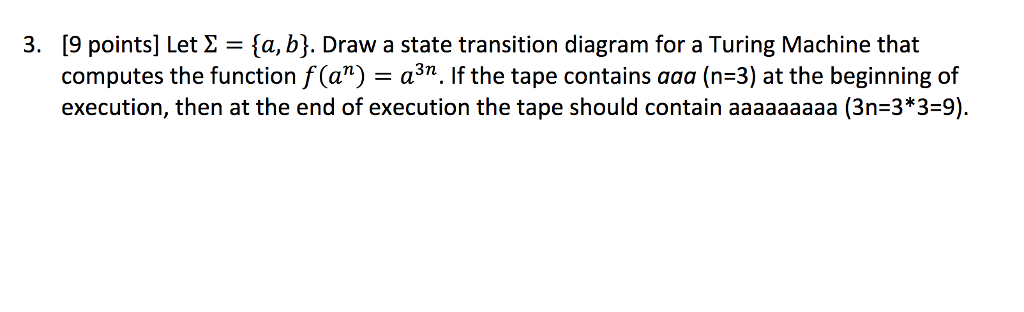Let sigma = {a, b}. Draw a state transition diagram for a Turing Machine that computes the function f(a^n) = a^3n. If the tape contains aaa (n=3) at the beginning of execution, then at the end of execution the tape should contain aaaaaaaaa (3n=3*3=9).